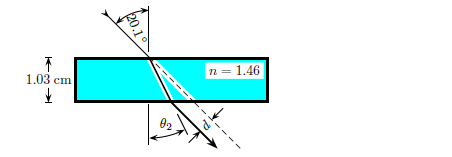# Problem: A narrow beam of light passes through a plate of glass with thickness 1.03 cm and a refractive index 1.46. The beam enters from air at an angle 20.1°. The goal is to calculate the deviation d of the ray as indicated in the figure. Find deviation d.

83% (59 ratings)
###### Problem Details

A narrow beam of light passes through a plate of glass with thickness 1.03 cm and a refractive index 1.46. The beam enters from air at an angle 20.1°. The goal is to calculate the deviation d of the ray as indicated in the figure. Find deviation d.Frequently Asked Questions

What scientific concept do you need to know in order to solve this problem?

Our tutors have indicated that to solve this problem you will need to apply the Refraction Of Light concept. You can view video lessons to learn Refraction Of Light. Or if you need more Refraction Of Light practice, you can also practice Refraction Of Light practice problems.

How long does this problem take to solve?

Our expert Physics tutor, Juan took 8 minutes and 52 seconds to solve this problem. You can follow their steps in the video explanation above.

What professor is this problem relevant for?

Based on our data, we think this problem is relevant for Professor Yao's class at TEXAS.